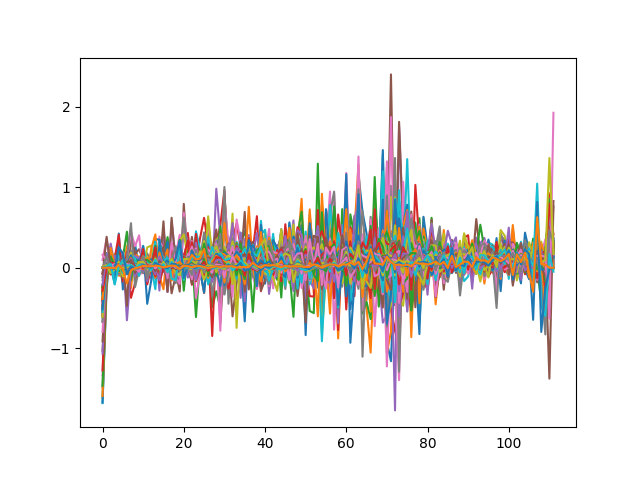# How to plot feature map

Hi, all.

I am currently trying to manipulate feature maps but first I want to visualize feature maps. I followed some instructions and get features I want. But when I plot them out, I dont think it is right. Attached is my code and result.

``````from torchvision import models
import foolbox
import torch
import torch.nn as nn
from matplotlib import pyplot as plt

image, label = foolbox.utils.imagenet_example(data_format="channels_first")
image = image/255
image = torch.from_numpy(image)
image = torch.unsqueeze(image, 0)

# remove last fully connected layer
resnet18 = models.resnet18(pretrained=True)
modules = list(resnet18.children())[:-9]
resnet18 = nn.Sequential(*modules)
for p in resnet18.parameters():

out = resnet18(image)
out = torch.squeeze(out, 0)
feature = out.numpy()

plt.plot(feature)
plt.show()
``````I guess `feature` is an activation map from a conv layer, is that correct? If so you could visualize each filter output (i.e. channel) using `plt.imshow`.

Oh I see. What a silly mistake I made. Thank you so much.